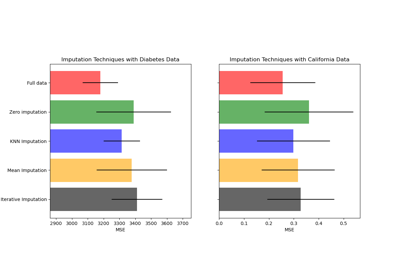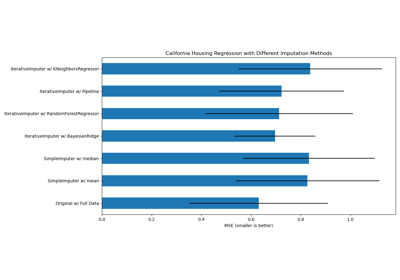# sklearn.impute.IterativeImputer¶

class sklearn.impute.IterativeImputer(estimator=None, *, missing_values=nan, sample_posterior=False, max_iter=10, tol=0.001, n_nearest_features=None, initial_strategy='mean', imputation_order='ascending', skip_complete=False, min_value=-inf, max_value=inf, verbose=0, random_state=None, add_indicator=False)[source]

Multivariate imputer that estimates each feature from all the others.

A strategy for imputing missing values by modeling each feature with missing values as a function of other features in a round-robin fashion.

Read more in the User Guide.

New in version 0.21.

Note

This estimator is still experimental for now: the predictions and the API might change without any deprecation cycle. To use it, you need to explicitly import enable_iterative_imputer:

>>> # explicitly require this experimental feature
>>> from sklearn.experimental import enable_iterative_imputer  # noqa
>>> # now you can import normally from sklearn.impute
>>> from sklearn.impute import IterativeImputer

Parameters:
estimatorestimator object, default=BayesianRidge()

The estimator to use at each step of the round-robin imputation. If sample_posterior=True, the estimator must support return_std in its predict method.

missing_valuesint or np.nan, default=np.nan

The placeholder for the missing values. All occurrences of missing_values will be imputed. For pandas’ dataframes with nullable integer dtypes with missing values, missing_values should be set to np.nan, since pd.NA will be converted to np.nan.

sample_posteriorbool, default=False

Whether to sample from the (Gaussian) predictive posterior of the fitted estimator for each imputation. Estimator must support return_std in its predict method if set to True. Set to True if using IterativeImputer for multiple imputations.

max_iterint, default=10

Maximum number of imputation rounds to perform before returning the imputations computed during the final round. A round is a single imputation of each feature with missing values. The stopping criterion is met once max(abs(X_t - X_{t-1}))/max(abs(X[known_vals])) < tol, where X_t is X at iteration t. Note that early stopping is only applied if sample_posterior=False.

tolfloat, default=1e-3

Tolerance of the stopping condition.

n_nearest_featuresint, default=None

Number of other features to use to estimate the missing values of each feature column. Nearness between features is measured using the absolute correlation coefficient between each feature pair (after initial imputation). To ensure coverage of features throughout the imputation process, the neighbor features are not necessarily nearest, but are drawn with probability proportional to correlation for each imputed target feature. Can provide significant speed-up when the number of features is huge. If None, all features will be used.

initial_strategy{‘mean’, ‘median’, ‘most_frequent’, ‘constant’}, default=’mean’

Which strategy to use to initialize the missing values. Same as the strategy parameter in SimpleImputer.

imputation_order{‘ascending’, ‘descending’, ‘roman’, ‘arabic’, ‘random’}, default=’ascending’

The order in which the features will be imputed. Possible values:

• 'ascending': From features with fewest missing values to most.

• 'descending': From features with most missing values to fewest.

• 'roman': Left to right.

• 'arabic': Right to left.

• 'random': A random order for each round.

skip_completebool, default=False

If True then features with missing values during transform which did not have any missing values during fit will be imputed with the initial imputation method only. Set to True if you have many features with no missing values at both fit and transform time to save compute.

min_valuefloat or array-like of shape (n_features,), default=-np.inf

Minimum possible imputed value. Broadcast to shape (n_features,) if scalar. If array-like, expects shape (n_features,), one min value for each feature. The default is -np.inf.

Changed in version 0.23: Added support for array-like.

max_valuefloat or array-like of shape (n_features,), default=np.inf

Maximum possible imputed value. Broadcast to shape (n_features,) if scalar. If array-like, expects shape (n_features,), one max value for each feature. The default is np.inf.

Changed in version 0.23: Added support for array-like.

verboseint, default=0

Verbosity flag, controls the debug messages that are issued as functions are evaluated. The higher, the more verbose. Can be 0, 1, or 2.

random_stateint, RandomState instance or None, default=None

The seed of the pseudo random number generator to use. Randomizes selection of estimator features if n_nearest_features is not None, the imputation_order if random, and the sampling from posterior if sample_posterior=True. Use an integer for determinism. See the Glossary.

If True, a MissingIndicator transform will stack onto output of the imputer’s transform. This allows a predictive estimator to account for missingness despite imputation. If a feature has no missing values at fit/train time, the feature won’t appear on the missing indicator even if there are missing values at transform/test time.

Attributes:
initial_imputer_object of type SimpleImputer

Imputer used to initialize the missing values.

imputation_sequence_list of tuples

Each tuple has (feat_idx, neighbor_feat_idx, estimator), where feat_idx is the current feature to be imputed, neighbor_feat_idx is the array of other features used to impute the current feature, and estimator is the trained estimator used for the imputation. Length is self.n_features_with_missing_ * self.n_iter_.

n_iter_int

Number of iteration rounds that occurred. Will be less than self.max_iter if early stopping criterion was reached.

n_features_in_int

Number of features seen during fit.

New in version 0.24.

feature_names_in_ndarray of shape (n_features_in_,)

Names of features seen during fit. Defined only when X has feature names that are all strings.

New in version 1.0.

n_features_with_missing_int

Number of features with missing values.

indicator_MissingIndicator

Indicator used to add binary indicators for missing values. None if add_indicator=False.

random_state_RandomState instance

RandomState instance that is generated either from a seed, the random number generator or by np.random.

SimpleImputer

Univariate imputer for completing missing values with simple strategies.

KNNImputer

Multivariate imputer that estimates missing features using nearest samples.

Notes

To support imputation in inductive mode we store each feature’s estimator during the fit phase, and predict without refitting (in order) during the transform phase.

Features which contain all missing values at fit are discarded upon transform.

Using defaults, the imputer scales in $$\mathcal{O}(knp^3\min(n,p))$$ where $$k$$ = max_iter, $$n$$ the number of samples and $$p$$ the number of features. It thus becomes prohibitively costly when the number of features increases. Setting n_nearest_features << n_features, skip_complete=True or increasing tol can help to reduce its computational cost.

Depending on the nature of missing values, simple imputers can be preferable in a prediction context.

References

Examples

>>> import numpy as np
>>> from sklearn.experimental import enable_iterative_imputer
>>> from sklearn.impute import IterativeImputer
>>> imp_mean = IterativeImputer(random_state=0)
>>> imp_mean.fit([[7, 2, 3], [4, np.nan, 6], [10, 5, 9]])
IterativeImputer(random_state=0)
>>> X = [[np.nan, 2, 3], [4, np.nan, 6], [10, np.nan, 9]]
>>> imp_mean.transform(X)
array([[ 6.9584...,  2.       ,  3.        ],
[ 4.       ,  2.6000...,  6.        ],
[10.       ,  4.9999...,  9.        ]])


Methods

 fit(X[, y]) Fit the imputer on X and return self. fit_transform(X[, y]) Fit the imputer on X and return the transformed X. get_feature_names_out([input_features]) Get output feature names for transformation. get_params([deep]) Get parameters for this estimator. set_params(**params) Set the parameters of this estimator. Impute all missing values in X.
fit(X, y=None)[source]

Fit the imputer on X and return self.

Parameters:
Xarray-like, shape (n_samples, n_features)

Input data, where n_samples is the number of samples and n_features is the number of features.

yIgnored

Not used, present for API consistency by convention.

Returns:
selfobject

Fitted estimator.

fit_transform(X, y=None)[source]

Fit the imputer on X and return the transformed X.

Parameters:
Xarray-like, shape (n_samples, n_features)

Input data, where n_samples is the number of samples and n_features is the number of features.

yIgnored

Not used, present for API consistency by convention.

Returns:
Xtarray-like, shape (n_samples, n_features)

The imputed input data.

get_feature_names_out(input_features=None)[source]

Get output feature names for transformation.

Parameters:
input_featuresarray-like of str or None, default=None

Input features.

• If input_features is None, then feature_names_in_ is used as feature names in. If feature_names_in_ is not defined, then the following input feature names are generated: ["x0", "x1", ..., "x(n_features_in_ - 1)"].

• If input_features is an array-like, then input_features must match feature_names_in_ if feature_names_in_ is defined.

Returns:
feature_names_outndarray of str objects

Transformed feature names.

get_params(deep=True)[source]

Get parameters for this estimator.

Parameters:
deepbool, default=True

If True, will return the parameters for this estimator and contained subobjects that are estimators.

Returns:
paramsdict

Parameter names mapped to their values.

set_params(**params)[source]

Set the parameters of this estimator.

The method works on simple estimators as well as on nested objects (such as Pipeline). The latter have parameters of the form <component>__<parameter> so that it’s possible to update each component of a nested object.

Parameters:
**paramsdict

Estimator parameters.

Returns:
selfestimator instance

Estimator instance.

transform(X)[source]

Impute all missing values in X.

Note that this is stochastic, and that if random_state is not fixed, repeated calls, or permuted input, results will differ.

Parameters:
Xarray-like of shape (n_samples, n_features)

The input data to complete.

Returns:
Xtarray-like, shape (n_samples, n_features)

The imputed input data.

## Examples using sklearn.impute.IterativeImputer¶Imputing missing values before building an estimator

Imputing missing values before building an estimatorImputing missing values with variants of IterativeImputer

Imputing missing values with variants of IterativeImputer